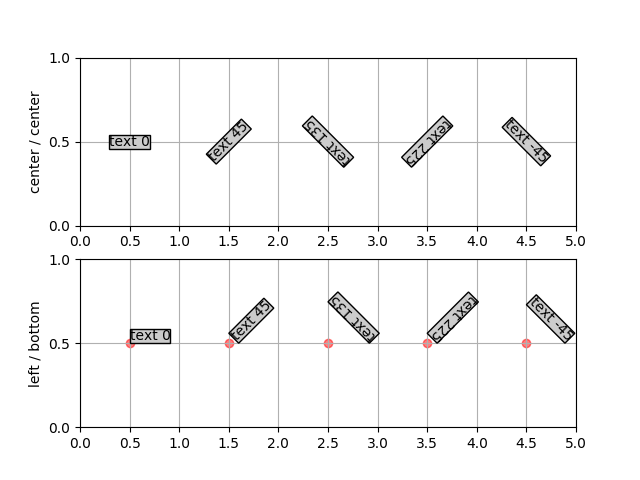# Default text rotation demonstration¶

The way Matplotlib does text layout by default is counter-intuitive to some, so this example is designed to make it a little clearer.

The text is aligned by its bounding box (the rectangular box that surrounds the ink rectangle). The order of operations is rotation then alignment. Basically, the text is centered at your x,y location, rotated around this point, and then aligned according to the bounding box of the rotated text.

So if you specify left, bottom alignment, the bottom left of the bounding box of the rotated text will be at the x,y coordinate of the text.

But a picture is worth a thousand words!import matplotlib.pyplot as plt
import numpy as np

ax.text(0.5, 0.5, 'text 0', props, rotation=0)
ax.text(1.5, 0.5, 'text 45', props, rotation=45)
ax.text(2.5, 0.5, 'text 135', props, rotation=135)
ax.text(3.5, 0.5, 'text 225', props, rotation=225)
ax.text(4.5, 0.5, 'text -45', props, rotation=-45)
for x in range(0, 5):
ax.scatter(x + 0.5, 0.5, color='r', alpha=0.5)
ax.set_yticks([0, .5, 1])
ax.set_xlim(0, 5)
ax.grid(True)

# the text bounding box
bbox = {'fc': '0.8', 'pad': 0}

fig, axs = plt.subplots(2, 1)

addtext(axs, {'ha': 'center', 'va': 'center', 'bbox': bbox})
axs.set_xticks(np.arange(0, 5.1, 0.5), [])
axs.set_ylabel('center / center')

addtext(axs, {'ha': 'left', 'va': 'bottom', 'bbox': bbox})
axs.set_xticks(np.arange(0, 5.1, 0.5))
axs.set_ylabel('left / bottom')

plt.show()


Keywords: matplotlib code example, codex, python plot, pyplot Gallery generated by Sphinx-Gallery